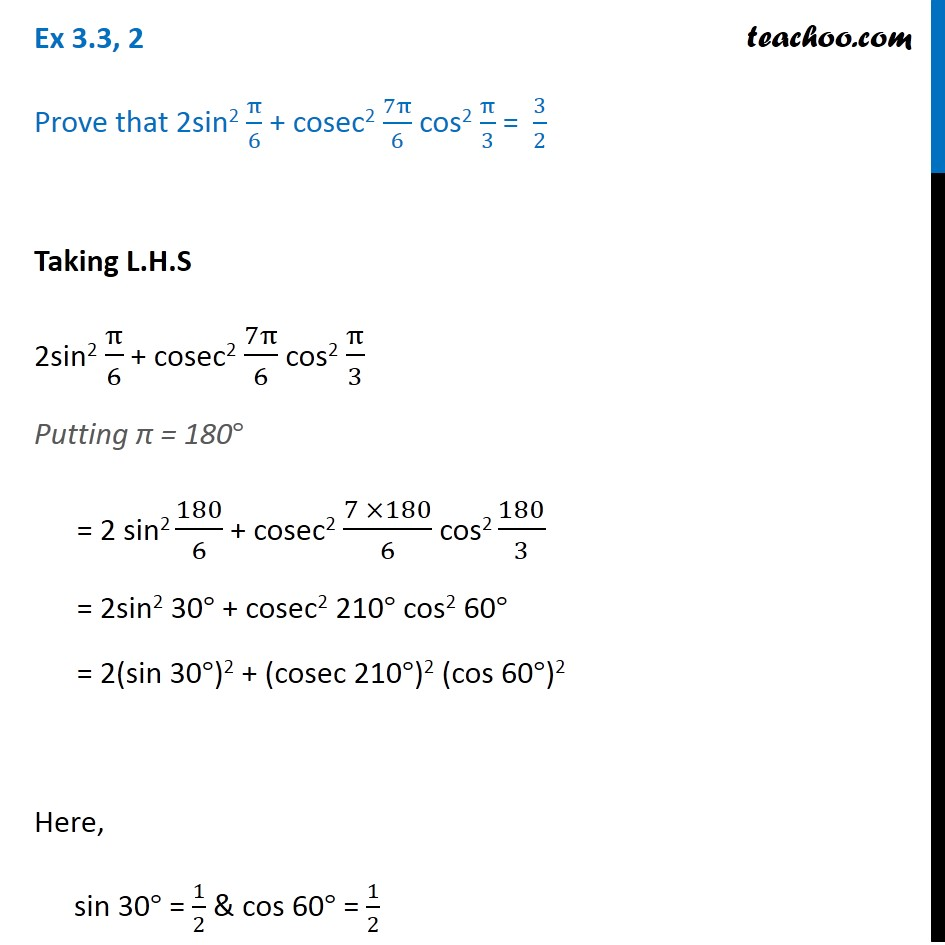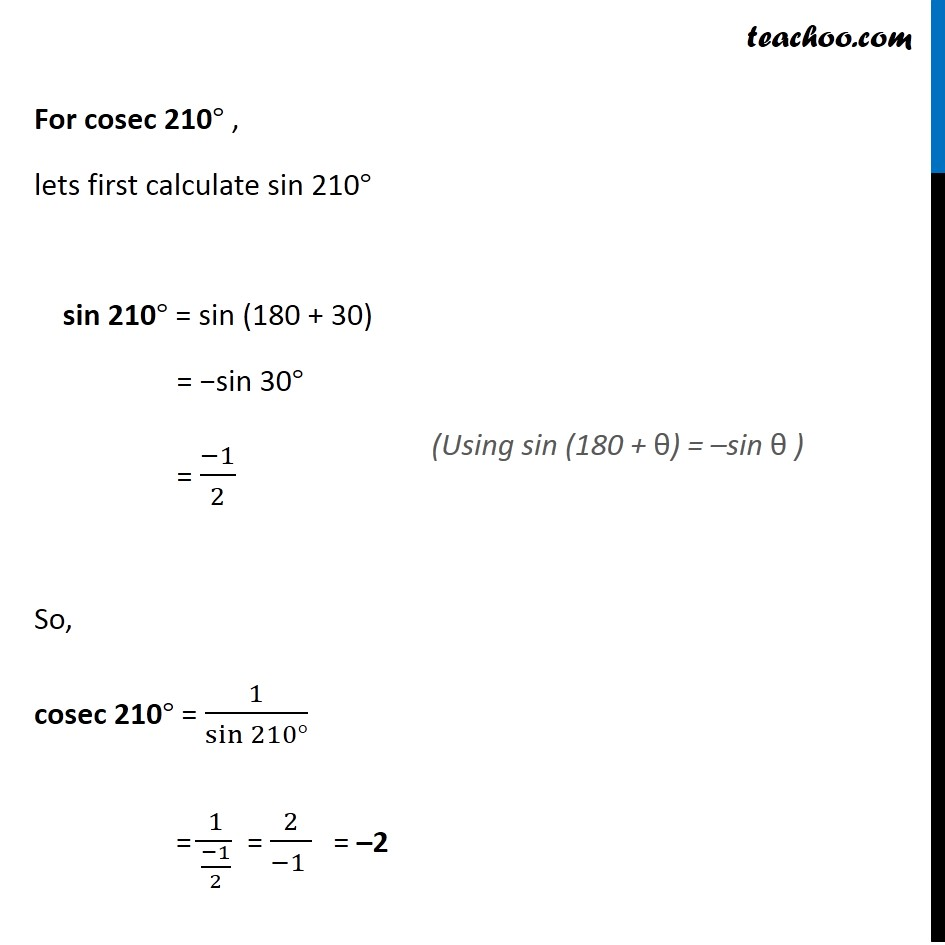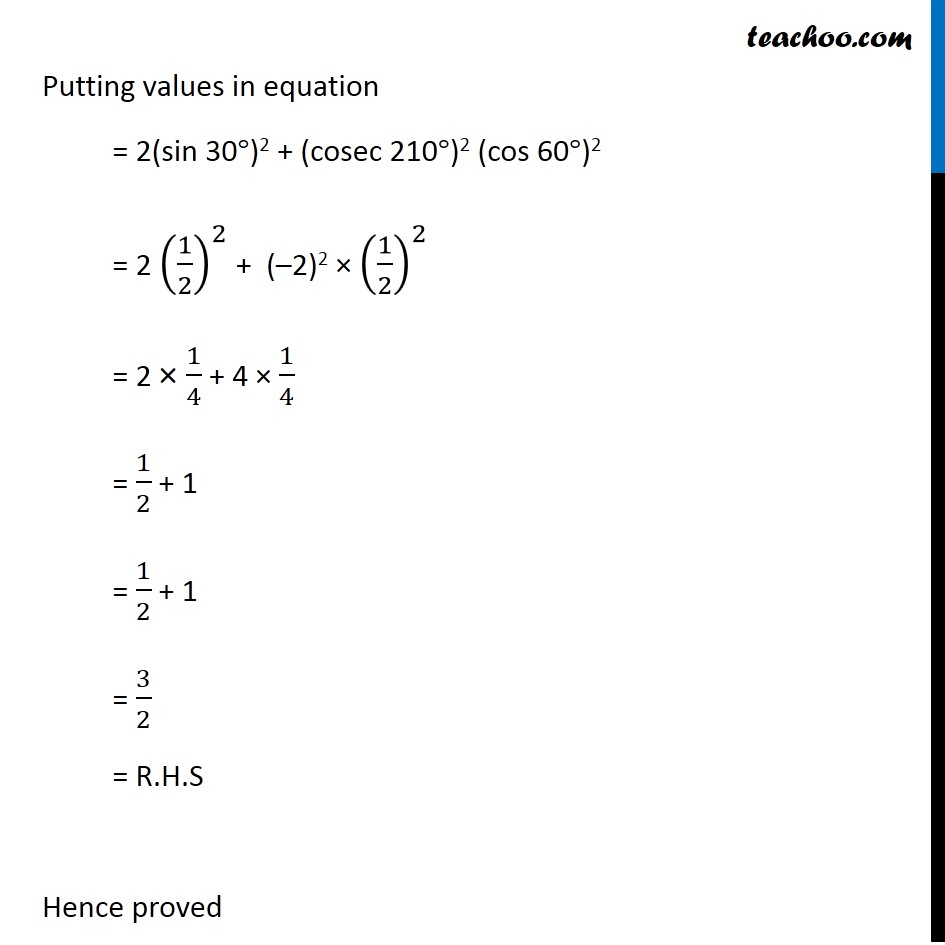Learn All Concepts of Chapter 2 Class 11 Relations and Function - FREE. Check - Trigonometry Class 11 - All ConceptsThere is some mistake in this Video. Please check images above.

1. Chapter 3 Class 11 Trigonometric Functions
2. Concept wise
3. Finding Value of trignometric functions, given angle

Transcript

Ex 3.3, 2 Prove that 2sin2 π/6 + cosec2 7π/6 cos2 π/3 = 3/2 Taking L.H.S 2sin2 π/6 + cosec2 7π/6 cos2 π/3 Putting π = 180° = 2 sin2 180/6 + cosec2 (7 ×180)/6 cos2 180/3 = 2sin2 30° + cosec2 210° cos2 60° = 2(sin 30°)2 + (cosec 210°)2 (cos 60°)2 Here, sin 30° = 1/2 & cos 60° = 1/2 For cosec 210° , lets first calculate sin 210° sin 210° = sin (180 + 30) = −sin 30° = (−1)/2 So, cosec 210° = 1/sin⁡〖210°〗 = 1/((−1)/2) = 2/(−1 ) = –2 (Using sin (180 + θ) = –sin θ ) Putting values in equation = 2(sin 30°)2 + (cosec 210°)2 (cos 60°)2 = 2 (1/2)^2 + (–2)2 × (1/2)^2 = 2 × 1/4 + 4 × 1/4 = 1/2 + 1 = 1/2 + 1 = 3/2 = R.H.S Hence proved

Finding Value of trignometric functions, given angle

About the AuthorDavneet Singh
Davneet Singh is a graduate from Indian Institute of Technology, Kanpur. He has been teaching from the past 9 years. He provides courses for Maths and Science at Teachoo.